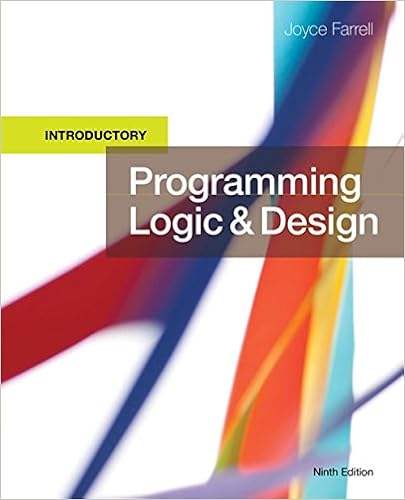# Design a retirement planning calculator for skulling

• Homework Help
• 53
• 95% (221) 210 out of 221 people found this document helpful

This preview shows page 31 - 36 out of 53 pages.

##### We have textbook solutions for you!
The document you are viewing contains questions related to this textbook.The document you are viewing contains questions related to this textbook.
Chapter 5 / Exercise 12
Programming Logic and Design, Introductory
FarrellExpert Verified
13. Design a retirement planning calculator for Skulling Financial Services. Allow a user to enter a number of working years remaining in the user’s career and the annual amount of money the user can save. Assume that the user earns three percent simple interest on savings annually. Output is a schedule that lists each year number in retirement starting with year 0 and the user’s savings at the start of that year. Assume that the user spends \$50,000 per year in retirement and then earns three percent interest on the remaining balance. End the list after 40 years or when the user’s balance is 0 or less, whichever comes first.
##### We have textbook solutions for you!
The document you are viewing contains questions related to this textbook.The document you are viewing contains questions related to this textbook.
Chapter 5 / Exercise 12
Programming Logic and Design, Introductory
FarrellExpert Verified
Programming Logic and Design, 8e Solutions 5-32 Pseudocode:
Programming Logic and Design, 8e Solutions 5-33 start Declarations num workingYrs num amtCanSave num balance num year num INTEREST_RATE = 0.03 num SPENDS = 50000 num MAX_YRS = 40 string PROMPT1 = “Enter the number of working years left in career >> ” string PROMPT2 = “Enter the amount that can be saved per year >> ” housekeeping detailLoop() endwhile finishUp() stop housekeeping() output PROMPT1 input workingYrs return detailLoop() output PROMPT2 input amtCanSave year = 0 balance = 0 while year < workingYrs balance = balance + amtCanSave balance = balance * (1 + INTEREST_RATE) year = year + 1 endwhile year = 0 while year <= MAX_YRS AND balance > 0 output year, balance balance = balance – SPENDING balance = balance * (1 + INTEREST_RATE) year = year + 1 endwhile return finishUp() output “End of program” return 14. Ellison Private Elementary School has three classrooms in each of nine grades, kindergarten through grade 8, and allows parents to pay tuition over the nine-month school year. Design the application that outputs nine tuition payment coupons for each of the 27 classrooms. Each coupon should contain the grade number (0 for kindergarten through 8), the classroom number (1 through 3), the month (1 through 9), and the amount of tuition due. Tuition for kindergarten is \$80 per month. Tuition for the other grades is \$60 per month times the grade level.
Programming Logic and Design, 8e Solutions 5-34 Answer: A sample solution follows Flowchart: Pseudocode: start Declarations num grade num room num month num tuition num MAX_GRADE = 8 num MAX_MONTH = 9
Programming Logic and Design, 8e Solutions 5-35 num MAX_ROOM = 3 num K_TUITION = 80 num TUITION_FACTOR = 60 housekeeping detailLoop() finishUp() stop housekeeping() grade = 0 return detailLoop() while grade <= MAX_GRADE if grade = 0 then tuition = K_TUITION else tuition = grade * TUITION_FACTOR endif room = 1 while room <= MAX_ROOM month = 1 while month <= MAX_MONTH output grade, room, month, tuition month = month + 1 endwhile room = room + 1 endwhile grade = grade + 1 endwhile return finishUp() output “End of program” return
•••